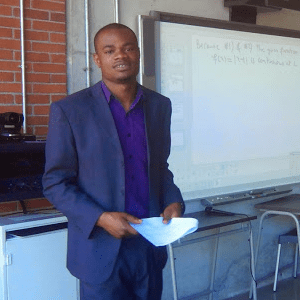## Noe Nshindi

Mathematics Bellville Noe N

### Teacher Info

I like to approach the Math lessons in four different ways:
Graphically using the coordinate planes
Numericaly using number table and number line
Analyticaly converting problems into equations and
Verbaly translating equation in the English language

Cost Starting from R300.00 for 60 min Mathematics 15 – 55 Intermediate, Advanced 1 – 3 Years

### Lesson Info

I always through those different approaches makes my student to understand the basic math concept.
For Maths problems, I show how to identify the unknown(s) and the given(s), how are them related through formula, properties, theorem etc, list the possible(s) solutions approach and select which one best suit the solver.

Share This